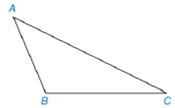Chapter 7.1, Problem 6EElementary Geometry For College St...

7th Edition
Alexander + 2 others
ISBN: 9781337614085

Solutions

Chapter
SectionElementary Geometry For College St...

7th Edition
Alexander + 2 others
ISBN: 9781337614085
Textbook Problem

In Exercises 3 to 8, use the drawing provided.Given: Obtuse ∆ A B C Construct: The perpendicular bisector of A C -

To determine

To construct:

The perpendicular bisector of AC- for the obtuse ABC as shown in the given figure.

Explanation

Definition:

Perpendicular bisector of a triangle is a line passing through the midpoint of the particular side of the triangle.

Calculation:

The given obtuse ABC is shown below:

To construct a bisector we follow the below procedure.

Step 1: Using the compass, take a length of two-third of the length of AC and take A as centre, draw an arc inside of the triangle.

Step 2: Similarly, take C as centre, draw an arc in the same side of arc drawn earlier to obtain a point D

Still sussing out bartleby?

Check out a sample textbook solution.

See a sample solution

The Solution to Your Study Problems

Bartleby provides explanations to thousands of textbook problems written by our experts, many with advanced degrees!

Get Started

Evaluate the expression sin Exercises 116. (23)2

Finite Mathematics and Applied Calculus (MindTap Course List)

Let f(x) = 1x+1 and g(x) = x2 + 1. Find the rules for (a) f + g, (b) fg (c) f t, and (d) g f.

Applied Calculus for the Managerial, Life, and Social Sciences: A Brief Approach

True or False: f(x)f(a)xa may be interpreted as the instantaneous velocity of a particle at time a.

Study Guide for Stewart's Single Variable Calculus: Early Transcendentals, 8th

Which curve is simple but not closed?

Study Guide for Stewart's Multivariable Calculus, 8th